# Optical Tweezers

Optical tweezers, is a single-molecule manipulation technique that can exert forces on particles (ranging from nanometers to microns) and at the same time measuring the displacement with sub-nanometer accuracy. Optical tweezing is simply the concentration of optical power in a small area. This concentration of power gives the ability to affect the motion of particles.

An optical tweezer system is developed to trap particles in gaseous environment. This system consists of three optical elements: Double Axicon (a), 45° mirror (b), and a Parabolic Mirror (c) (Figure 1). Figure 2 shows a schematic of the complete setup in which observation tools are also apparent. Using this setup, spherical fused silica particles with a diameter of 10µm were optically trapped (Figure 3).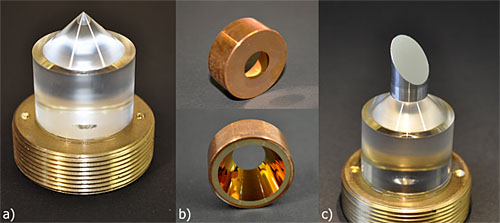Figure 1. Optical elements of optical tweezers system for particle trapping in gaseous surrounding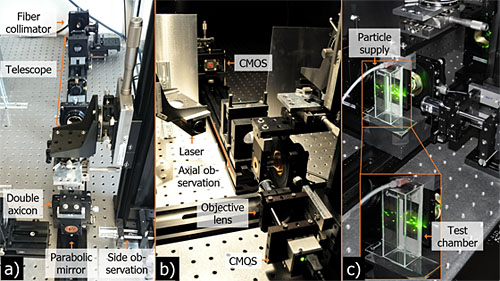Figure 2. Optical setup for the optical tweezers for gaseous surrounding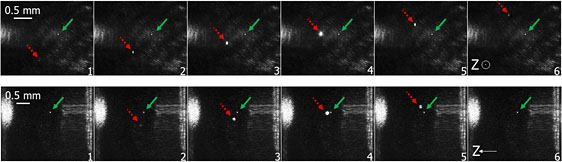Figure 3. Optical trapping of silica fused particle in gaseous environment by means of optical tweezers. The green arrow points at the trapped particle.

In addition, a simulation tool is also developed for the theoretical investigation of optical forces and torque acting on microscopic objects, based on the Geometrical optics approach. The objects can have the form of cells, beads and droplets (ellipsoids). This tool can be used to study effect of parameters such as NA, intensity distribution, refractive index of the particles and the surrounding, on the optical forces. This tool can be used to optimize an optical trapping system for specific applications in which output intensity, NA, aberrations are known. Figure 4 and 5 shows the simulation process for the optical trapping system developed for gaseous environment.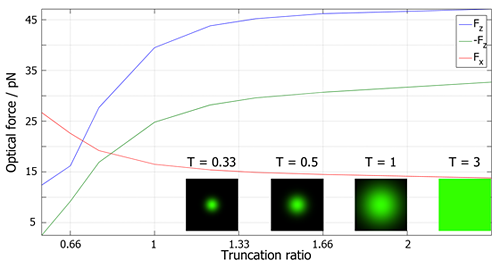Figure 4. Optical Force as a function of the Truncation Ratio of a Gaussian Beam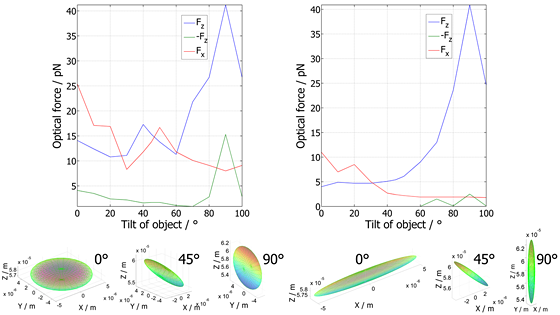Figure 5. Optical Forces acting on Oblate (left) and needle (right) shaped PMMA particles in liquid at title angles around the Y axis

Optical tweezers are used in a variety of applications such as Single-molecule biophysics, Cell biology, Spectroscopy, optofluidics and lab-on-a-chip, colloid science, microchemistry, aerosol science, plasmonics, laser cooling, and etc.

This work has been the major work of a former Ph.D. student, Ronald Kampmann, and in collaboration with Prof. S. Sinzinger from TECHNISCHE UNIVERSITÄT ILMENAU (http://www.tu-ilmenau.de/to/).

Further Information: